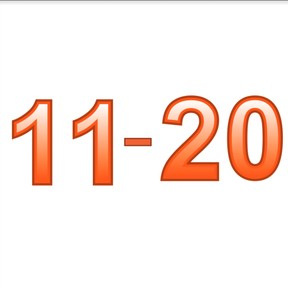Know numbers to 20

# Know numbers to 20

Know numbers to 20

No account needed.8,000 schools use Gynzy92,000 teachers use Gynzy1,600,000 students use Gynzy

## General

Students recognize numbers to 20 on the basis of their spoken name, and can write down the accurate number. Students can write the number, say the word, and write the number in words.

K.CC.A.3

## Relevance

Discuss with students that numbers are all around us and that you need to learn to recognize them. An example is when you travel with the bus, you want to be sure you take the right bus.

## Introduction

Students practice recognizing the numbers to 20 by circling pairs of numbers.

## Development

The teacher points at the numbers from 11 to 20 and says their names aloud. Ask the students to repeat the names after you. Next the numbers are in random order. Point at a number and ask the students to say the number aloud. Explain how the number is written. Next say one number and ask students to write the answers down.

Check that students have understood by asking the following questions:
Where might you see or find numbers?
What kind of example can you give? (calendar, jersey numbers for sports, digital clock)
Which number is it? You can choose to write a few numbers on the board and ask the students to name them.

## Guided Practice

First students are asked to link a spoken number to a visual (numerical) number and then to write the number for a spoken number.

## Closing

Discuss with students why it is important to recognize numbers to 20. Numbers are everywhere. An example is if you travel by bus, you want to be sure you are on the right bus. Check that students understand by asking them to practice 11-20 in pairs with number cards. The first student lays down a number and the second must name the number. They take turns.

## Teaching Tip

Students who still have trouble can practice with speaking and writing numbers to 10. The students who are ready for more challenge can work on numbers to 30.

## Instruction materials

Number cards 11 - 20.

### The online teaching platform for interactive whiteboards and displays in schools

• Save time building lessons

• Manage the classroom more efficiently

• Increase student engagement